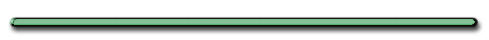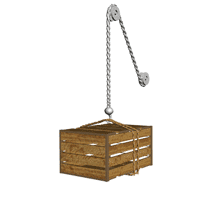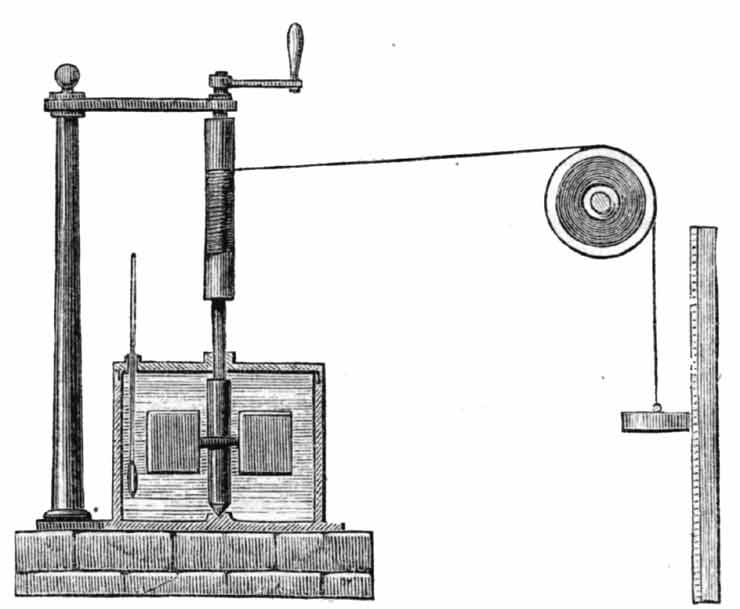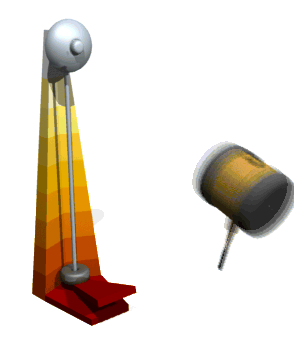Homehttp://www.ignitelearning.com/ENERGY PROBLEMS

When work is done on or by a system

Approximately how much kinetic energy
does the green box acquire?

[ Flash ]

PE = Just under 600 Nm

 Ex 1) An object slides across a horizontal table which changes its KE from 20 J to 18 J in 2 sec. What work does the object do against friction?

Ex 1) An object slides across a horizontal table which changes its kinetic energy from 20 J to 18 J in 2 sec. What work does the object do against friction?

W = 2 J

 **Work against friction increases an object's Internal Energy** (makes it hotter) Friction is a nonconservative force (doesn't store energy) (can't get back)

Conservation of EnergyHarper's New Monthly Magazine, No. 231, August, 1869.

Penn State Schuylkill

Work Energy Relationship

The work done on or by a system

= ΔKE + ΔPE + WfWf - work done against friction

If ΔPE of yellow box = 30 J,
how much work did the green box do on it?

 Work done by the ball on the floor changes the ball's mechanical energy

 When the WORK equation  doesn't WORK, Use an ENERGY equation. When an ENERGY equation  doesn't WORK,   Use the WORK equationEnergy ProblemsEnrichment

Superfluids

The strange behavior of liquid helium

BBC